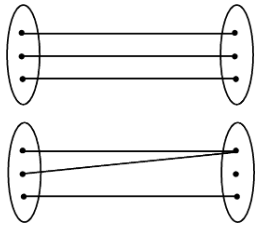# Type of Functions

## Type of Functions

### (a) One-One (Injective)

If x1, x2 ∈ A

then f(x1) = f(x2) ⇒ x1 = x2

and x1 ≠ x2 ⇒ f(x1) ≠ f(x2)

### (b) Many-One

If x1, x2 ∈ A, f(x1) = f(x2) ⇒ x1 ≠ x2

### (c) Onto (Surjective) and Into

If f(A) = B i.e. Range = co-domain then the function is ONTO (Surjective) and If f(A) ⊂ B i.e. Range ⊂ co domain then the function is INTO.

One-One onto functions are also called bijective functions.